×#### Thank you for registering.

One of our academic counsellors will contact you within 1 working day.

Click to Chat

1800-1023-196

+91-120-4616500

CART 0

• 0

MY CART (5)

Use Coupon: CART20 and get 20% off on all online Study Material

ITEM
DETAILS
MRP
DISCOUNT
FINAL PRICE
Total Price: Rs.

There are no items in this cart.
Continue Shopping```Electrode Potential

Table of Content

What is Electrode Potential?

Standard Electrode Potential

Reference Electrode

Measurement of Electrode Potential

Determination of Standard Electrode Potential of Zn/Zn2+ Electrode

Determination of Standard Electrode Potential of Cu2+/Cu, Electrode

Some Other Reference Electrodes

Calomel Electrode

Ferrous – Ferric Electrode

Quin – Hydrone Electrode

Metal – Metal ion Electrode

Amalgam Electrode

Related Resources

What is Electrode Potential?When a metal is placed in a solution of its ions, the metal acquires either a positive or negative charge with respect to the solution. On account of this, a definite potential difference is developed between the metal and the solution. This potential difference is called electrode potential.For example, when a plate of zinc is placed in a solution having Zn2+ ions, it becomes negatively charged with respect to solution and thus a potential difference is set up between zinc plate and the solution. This potential difference is termed the electrode potential of zinc.

Similarly, when copper is placed in a solution having Cu2+ ions, it becomes positively charged with respect to solution. A potential difference is set up between the copper plate and the solution. The potential difference thus developed is termed as electrode potential of copper. The potential difference is estab­lished due to the formation of electrical double layer at the

Following two changes occur when a metal rod is dipped in its salt solution,

(a) Oxidation: Metal ions pass from the electrode into solution leaving an excess of electrons and thus a negative charge on the electrode.The conversion of metal atoms into metal ions by the attractive force of polar water molecules.

M →  Mn + ne-

The metal ions go into the solution and the electrons remain on the metal making it negatively charged. The tendency of the metal to change into ions is known as electrolytic solution pressure.

(b) Reduction: Metal ions in solution gain electrons from the electrode leaving a positive charge on the electrode. Metal ions start depositing on the metal surface leading to a positive charge on the metal.

Mn+ +  ne- →  M

In the beginning, both these changes occur with different speeds but soon an equilibrium is established.

M Mn+ + ne-

In practice, one effect is greater than the other,

If first effect is greater than the second, the metal acquires a negative charge with respect to solution and

If the second is greater than the first, it acquires positive charge with respect to solution, thus in both the cases a potential difference is set up.

The magnitude of the electrode potential of a metal is a measure of its relative tendency to lose or gain electrons, i.e., it is a measure of the relative tendency to undergo oxidation (loss of electrons) or reduction (gain of electrons).Depending on the nature of the metal electrode to lose or gain electrons, the electrode potential may be of two types:

Oxidation potential: When electrode is negatively charged with respect to solution, i.e., it acts as anode. Oxidation occurs.

M →  Mn+ + ne-

Reduction potential: When electrode is positively charged with respect to solution, i.e., it acts as cathode. Reduction occurs.

Mn+ + ne- →  M

It is not possible to measure the absolute value of the single electrode potential directly. Only the difference in potential between two electrodes can be measured experimentally.  It is, therefore, necessary to couple the electrode with another electrode whose potential is known. This electrode is termed as reference electrode. The EMF of the resulting cell is measured experimentally.

The EMF  of the cell is equal to the sum of potentials on the two electrodes.

Emf of  the cell = EAnode + ECathode  = Oxidation potential of anode + Reduction potential of cathode

Knowing the value of reference electrode, the value of other electrode can be determined.

Standard Electrode Potential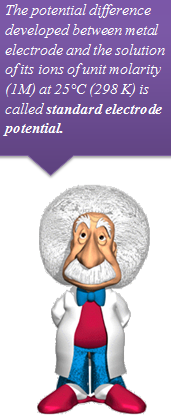In order to compare the electrode potentials of various electrodes, it is necessary to specify the concentration of the ions present in solution in which the electrode is dipped and the temperature of the half-cell.

The potential difference developed between metal electrode and the solution of its ions of unit molarity (1M) at 25°C (298 K) is called standard electrode potential.

According to the IUPAC convention, the reduction potential alone be called as the electrode potential (EO), i.e., the given value of electrode potential be regarded as reduction potential unless it is specifically mentioned that it is oxidation potential.

Standard reduction potential of an electrode means that reduc­tion reaction is taking place at the electrode. If the reaction is reversed and written as oxidation reaction, the numerical value of electrode potential will remain same but the sign of standard potential will have to be reversed. Thus

Standard reduction potential = - (Standard oxidation potential)

or

Standard oxidation potential = - (Standard reduction potential)

Reference Electrode

( Standard Hydrogen Electrode, SHE)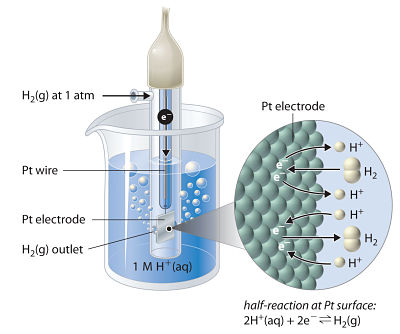Hydrogen electrode is the primary standard electrode.

It con­sists of a small platinum strip coated with platinum black as to adsorb hydrogen gas.

A platinum wire is welded to the platinum strip and sealed in a glass tube as to make contact with the outer circuit through mercury.

The platinum strip and glass tube is surrounded by an outer glass tube which has an inlet for hydrogen gas at the top and a number of holes at the base for the escape of excess of hydrogen gas.

The platinum strip is placed in an acid solution which has H+ ion concentration 1 M.

Pure hydrogen gas is circulated at one atmospheric pressure.

A part of the gas is adsorbed and the rest escapes through holes. This gives an equilibrium between the adsorbed hydrogen and hydrogen ions in the solution.

H2  2H+ + 2e-

The temperature of the cell is maintained at 250C. By international agreement the standard hydrogen electrode is arbitrarily assigned a potential of exactly ± 0.000 . .. volt.

The hydrogen electrode thus obtained forms one of two half-cells of a voltaic cell. When this half-cell is connected with any other half-cell, a voltaic cell is constituted. The hydrogen electrode can act as cathode or anode with respect to other electrode.

SHE half reaction

Electrode potential

H2 →  2H+ + 2e-

0.0 V (Anode)

2H+ + 2e- →  H2

0.0 V (Cathode)

Measurement of Electrode Potential

The measurement of electrode potential of a given electrode is made by constituting a voltaic cell, i.e., by connecting it with a standard hydrogen electrode (SHE) through a salt bridge.

1 M solution is used in hydrogen half-cell and the temperature is maintained at 25oC.The EMF of the cell is measured either by a calibrated potentiometer or by a high resistance voltmeter, i.e., a valve voltmeter. The reading of the voltmeter gives the electrode potential of the electrode in question with respect to the hydrogen electrode. The standard electrode potential of a metal may be determined as it is the potential difference in volt developed in a cell consisting of two electrodes: the pure metal is contact with a molar solution of one of its ions and the standard hydrogen electrode.

Refer to the following video for standard hydrogen electrode

Determination of Standard Electrode Potential of Zn/Zn2+ Electrode

A zinc rod is dipped in 1 M zinc sulphate solution. This half-cell is combined with a standard hydrogen electrode through a salt bridge.

Both the electrodes are con­nected with a voltmeter.

The deflection of the voltmeter indicates that current is flowing from hydrogen electrode to metal electrode or the electrons are moving from zinc rod to hydrogen electrode.

The zinc electrode acts as an anode and the hydrogen electrode as cathode and the cell can be represented as

Cell Representation

Oxidation half reaction

Reduction half reaction

Zn|Zn2+ (aq)/Anode(-) || 2H(aq)| H2 (g)/Cathode (+)

Zn →  Zn2+ + 2e-

2H+ + 2e- →  H2↑The EMF of the cell is 0.76 volt

ECell = EoAnode + EoCathode

0.76 =  EoAnode + 0 or EoAnode = +0.76 V

As the reaction on the anode is oxidation, i.e.,

Zn →  Zn2+ + 2e,

EoAnode is the standard oxidation potential of zinc. This potential is given the positive sign.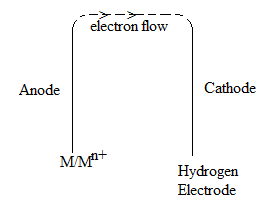Eoox (Zn/Zn2+) = +0.76 volt

So standard reduction potential of Zn, i.e., Eo (Zn/Zn2+)

= Eoox = -(+0.76)

= -0.76 volt

The EMF of such a cell gives the positive value of standard oxidation potential of metal M. The standard reduction potential (Eo) is obtained by reversing the sign of standard oxidation potential.

Determination of Standard Electrode Potential of Cu2+/Cu, Electrode:

A copper rod is dipped in 1 M solution of CuSO4. It is combined with hydrogen electrode through a salt bridge. Both the electrodes joined through a voltmeter. The deflection of the voltmeter indicates that current is flowing from copper electrode towards hydrogen electrode, i.e., the electrons ate moving from hydrogen to copper electrode. The hydrogen electrode acts as an anode and the copper electrode as a cathode. The cell can be represented as

Cell Representation

Oxidation half reaction

Reduction half reaction

H2 (g)|2H+ (aq) ||Cu2+ (aq) |Cu

H2 →  2H+ + 2e-

Cu2+ 2e- →  Cu

The EMF of the cell is 0.34 volt.

EoCell = EoAnode + EoCathode

0.34 = 0 + EoCathode

Since the reaction on the cathode is reduction, i.e.,

Cu2+ + 2e- →  Cu, EoCathode  is the standard reduction potential of copper. This is given the +ve sign.

E°, i.e., standard reduction potential of Cu2+/Cu = 0.34 volt

So E°x (standard oxidation potential of copper) = -0.34 volt

The EMF of such a cell gives positive value of reduction potential of metal electrode. The standard oxidation potential of this electrode is obtained by reversing the sign of standard reduction potential.

The EMFof such a cell gives the positive value of standard oxidation potential of metal M. The standard reduction potential (E°) is obtained by reversing the sign of standard oxidation potential.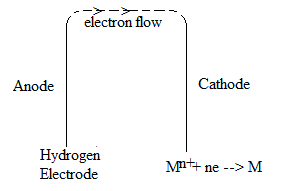It is thus concluded that at the metal electrode which acts as anode with respect to hydrogen electrode (cathode), the reduc­tion potential is given the minus sign and at the metal electrode which acts as cathode with respect to hydrogen electrode (anode), the reduction potential is given the positive sign.

The standard electrode potentials (oxidation or reduction) of various elements can be measured by combining the electrode in question with a standard hydrogen electrode and measuring the emf of the cell constituted.

Some Other Reference Electrodes

Since a standard hydrogen electrode is difficult to prepare and maintain, it is usually replaced by other reference electrodes, which are known as secondary reference electrodes. These are convenient to handle and are prepared easily. Two important secondary reference electrodes are described here.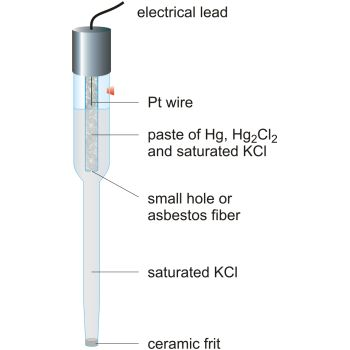Calomel Electrode

It consists of mercury at the bot­tom over which a paste of mercury-mercurous chloride is placed. A solution of potassium chloride is then placed over the paste. A platinum wire sealed in a glass tube helps in making the electrical contact. The electrode is connected with the help of the side tube on the left through a salt bridge with the other electrode to make a complete cell.

The potential of the calomel electrode depends upon the concentration of the potassium chloride solution. If potassium chloride solution is saturated, the electrode is known as saturated calomel electrode (SCE) and if the potassium chloride solution is 1 N, the electrode is known as normal calomel electrode (NCE) while for 0.1 N potassium chloride solution, the electrode is referred to as decinormal calomel electrode (DNCE). The electrode reaction when the electrode acts as cathode is:

1/2 Hg2Cl2 + e- Hg + Cl-

The reduction potentials of the calomel electrodes on hydrogen scale at 298K are as follows:

Concentration of KCl

Reduction Potential

Saturated KCl

0.2415 V

1.0NKCl

0.2800 V

0.1NKCl

0.3338 V

The electrode potential of any other electrode on hydrogen scale can be measured when it is combined with calomel electrode. The emf of such a cell is measured. From the value of electrode potential of calomel electrode, the electrode poten­tial of the other electrode can be evaluated.

Ferrous – Ferric Electrode

An example is a Pt wire dipping in a solution containing ferrous and ferric ions. Such a cell is described as: Pt | Fe2+ (C1), Fe3+ (C2)
The comma is used to separate two chemical species in the same solution. The electrode reaction is

Fe3+ + e– →   Fe2+

The function of a Platinum wire is to pick up the electrons and provide electrical contact to the electrode.

Quin – Hydrone Electrode

Oxidation reduction electrodes can also be made with organic molecules that can exist in two different oxidation states.

A generally used material of this type is related to important biochemical oxidation – reduction reactions, is the system of hydroquinone, which can form the oxidation reduction system, between quinone (Q) and hydroquinone (QH2).The presence of a Pt electrode in a solution containing these two species again clearly provides an electrode that can donate or accept electrons.

If hydro – quinone is represented by QH2 and quinone by Q, the electrode is abbreviated as
Pt.|QH2,Q,H+ (C)

This electrode is called a quin-hydrone electrode, because of the charged complex that is formed between QH2 and Q.

Metal – Metal ion Electrode

An example of this is a metallic silver electrode in an AgNO3 solution. The electrode is represented as Ag | Ag+ (C) and the electrode reaction is Ag+ + e–  →  Ag.

Note:   Very active metals react directly with water itself and cannot be used for such electrodes.

Another example is Silver-silver chloride electrode

This is another widely used reference electrode. It is reversible and stable and can be combined with cells containing chlorides without insetting liquid junctions.

Silver chloride is deposited electrolytically on a silver or platinum wire and it is then immersed in a solution containing chloride ions. Its standard electrode potential with respect to the standard hydrogen electrode is 0.2224 V at 298 K. the electrode is represented as:  Ag|AgCl|Cl- and the electrode reaction is, AgCl+e-→  Ag + Cl-Amalgam Electrode

In a variation of  the previous electrode, the metal is in the form of an amalgam, i.e., it is dissolved in mercury, rather than in the pure form. Electrical contact is made by a Pt wire dipping into the amalgam pool. The reaction is the same as in the metal–metal ion electrode, with the Hg playing no role. A sodium amalgam electrode is represented as Na [ in Hg at C1] | Na+ (C2).Question 1: Which of the following equations represents calomel electrode?

a. Hg2Cl2 + e- Hg + Cl-

b. AgCl (s) + e–  →   Ag + Cl–

c.  Fe3+ + e– →   Fe2+

d.  H2   2H+ + 2e-

Question 2: Magnitude of electrode potential does not depend on.

a. Pressure

b. Temperature

c. Concentration

d.  Nature of electrode

Question 3: In which of the following elements is not a component of  SHE?

a. Platinum

b. Hydrogen

c. Mercury

d. Gold

Question 4: SHE can be used as..

a. only anode

b. only cathode

d. both anode and cathode

c. neithet anode nor cathode

Question 5:  E° value for  Cu2+/Cu  volt is

a. 0.34 V

b. 0.58 V

c. 1.02 V

d. 1.51 VQ.1

Q.2

Q.3

Q.4

Q.5

a

a

d

d

a

Related Resources

Refer to Electrolysis and Electrolytic Cell

You can get the knowledge of Useful Books of Chemistry

To read more, Buy study materials of Electrochemistry comprising study notes, revision notes, video lectures, previous year solved questions etc. Also browse for more study materials on Chemistry here.
```### Course Features

• 731 Video Lectures
• Revision Notes
• Previous Year Papers
• Mind Map
• Study Planner
• NCERT Solutions
• Discussion Forum
• Test paper with Video Solution